# Learning Data Science with R: Variables, Data types and Operators

Just like other programming languages, the basic building blocks of the R language are its variables, data types and operators. Without knowing the basics, it is quite impossible to excel at R and eventually data science. So, let’s start with the basics.

Variables in R

In simple language, a variable is a temporary data storage space. A variable can store any type of data in R. There is no need to define the data type like in some programming language such as C, C++, Java , C#, etc. Variables in R are dynamically type allocated just like Python.

Syntax:

`variable_name = value `

(Variable assignment is discussed below in detail.)

Here, the variable name can be anything of your choice which starts with either alphabet, or dot(.). The variable should not start with number or underscore and end with %. The legal variable name includes `var1`, `var_3`, `.var_name`, `var.name`. Some of the invalid variable names are `_var2`, `1var`, `_var_name`, `.1var`.

Data Types in R

There are various data types used in R. Some of the common data types used are:

• Numeric : Numeric data is the most common data type used in R. As the name suggests it consists of numbers: both decimal and floating point. Example: 8, 3892.72, 190
Variable assignment :` num1 <- 5`
• Character : Character data type encompasses the alphabets, words and sentences. The value is enclosed within double quotation marks as :
`char1 = “Sam”` , `char2 <- "Hello there"`
• Logical : Logical data type consists of Boolean data TRUE and FALSE. Eg.
`log1 = TRUE `, `log2 = FALSE`
• Complex : As the name suggests the complex data type consists of complex numbers. Eg.
`comp1 = 5+9i` , `comp2 = -9–5i`

Similarly, there are other data types as well such as Integer and Raw. But these data types are not used much.

The data type of the variable can be checked with the following syntax:

`class(num1) "numeric"`

(Output was obtained using RStudio Console.)

Operators in R

An operator is used to command a compiler to perform a certain mathematical or logical operation on the operands. There are 5 commonly used operators in R. They are:

• Assignment operator: Assignment operator is used to assign value(s) to the variables. There are 3 ways of assigning a value in R. We can use either `=`, `<- `or `->`. Similarly, `->>` and `<<-` are also used.
`var1 = 3var1 <- 69 -> var1`
• Arithmetic operator: Arithmetic operators are used to perform the major arithmetic operations such as addition, subtraction, multiplication and division. The operators used are: `+`, `-` , `*` and `/` .
`num1 = 100num2 = 10num1+num2 110num1-num2 90num1*num2 1000num1/num2 10`
• Relational operator: Relation operator is used to compare two or more values or variables. Some commonly used operators are: `<` , `>` , `==` , `!=` , `<=` and `>=` .
`num1 = 100num2= 10num1 == num2 FALSEnum1 < num2 FALSEnum1 != num2 TRUE`
• Logical operator: Logical operators are used to perform logical operations such as logical AND and logical OR. The operators used are : `|` , `&` , `!` .
`log1 = TRUElog2 = FALSE#OR operationlog1 | log1 TRUElog1 | log2 TRUElog2 | log2 FALSE# AND operationlog1 & log2 FALSE#NOT operation!log1 FALSE`

There are other miscellaneous operators used such as: `: `, `%in% `, `%*% `.

I’ve used RStudio to perform the calculations discussed above. A brief article regarding the usability and setup will come in a few days.

This is the second article in the series Learning Data Science with R . I’ve written what I have learned. Some information may not be complete so refer to other sources as well.

--

--

--

## More from Dhiraj Raut

Learning and Sharing

Love podcasts or audiobooks? Learn on the go with our new app.

## Scrimba release notes — October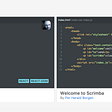## AWS SageMaker and GitHub Integration## Benefits of Using Cloudflare on Your Site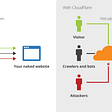## Setting up your NAS Box, 2021 Edition, Part 2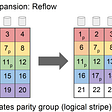## The Benefits of Software Standards## MySQL: Init GORM from YAML with rk-boot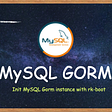## Dhiraj Raut

Learning and Sharing

## How to install, setup and get started with RStudio IDE on your Windows 10 device…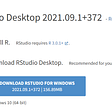## Introductory Statistics (Personal Note 1)— Data